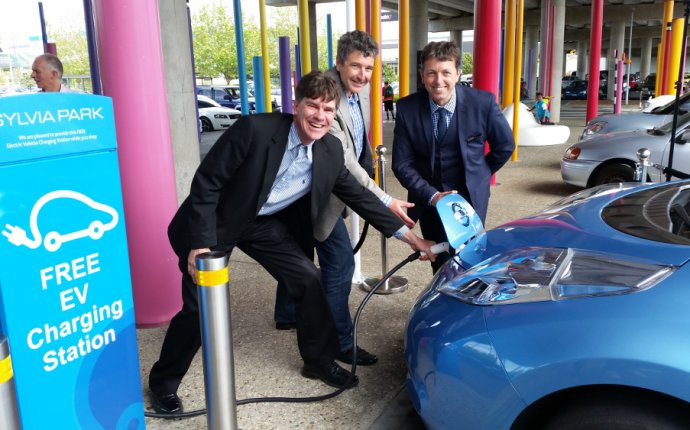## Car Battery charging Cost

Car Charging Socket / August 2, 2016

Depending where you live, electricity is rather cheap. Here's some math and some definitions, to take some of the mystery out of car batteries.

Amp-hours: Car batteries have a range of capacities, measured in Amp-hours. The Ampère is the unit of electrical flow: 1 Amp is a flow of 1 Coulomb (unit of electrical charge) per second. An hour is 3600 seconds, an Amp-hour is a flow of 1 Amp for 1 hour, so an Amp-hour is 1 Coulomb/second x 3600 seconds, so a total electrical charge of 3600 Coulombs.

Battery rating: Car batteries have a range of ratings, lets pick 45 Amp-hours. Since an Amp-hour is an electrical charge of 3600 Coulombs, this means that a car battery with a rating of 45 Amp-hours has an electrical charge of 45 x 3600 Coulombs, or 162, 000 Coulombs.

Car Battery Electrical Energy: Electricity from a typical car battery is delivered at 12 Volts. The Volt is the unit of electrical potential and is equivalent to a potential of 1 Joule (unit of energy) per Coulomb. So, at 12 Volts, our 162, 000 Coulombs car battery is storing 12 Joules/Coulomb x 162, 000 Coulombs, or 1, 944, 000 Joules.

kW-hours: Home electricity is usually billed in kW-hours. A kW (kiloWatt) is the unit for the rate of energy consumption, equivalent to 1 kJ (1000 Joules) per second. So, 1 kW-hour is a rate of consumption of 1 kW for 1 hour, or 1 kJ/s x 1 hour. An hour is 3600 seconds, so 1 kJ/s x 3600 s means a kW-hour is a consumption of 3600 kJ.

kW-hours needed to charge your battery: Assuming 100% efficiency (which will not be the case), the car battery will use 1, 944, 000 Joules to get a full charge, or 1, 944 kiloJoules (a simple unit conversion). Since each kW-hour represents 3600 kiloJoules, a full charge for our car battery represents 1, 944 kJ x 1 kW-hour/3600 kJ, or 0.54 kW-hours.

Cost of home electricity: This varies greatly with location, so let's pick \$0.10/kW-hour. Our cost to fully charge our battery (again assuming 100% efficiency, which won't happen) is 0.54 kW-hours x \$0.10/kW-hour, or \$0.05.

Source: mechanics.stackexchange.com